# Examples on Domains and Ranges of Functions Set 3

Go back to  'Functions'

Example- 12

Find the range of the following functions, assuming real functions.

 (a) $$f(x) = x$$ (b) $$f(x) = {x^2} + 1$$ (c) $$f(x) = \sqrt {\sin x}$$ (d) $$f(x) = [\sin x]$$ (e) f(x) = \begin{align}\frac{1}{{[x]}}\end{align} (f) f(x) =\begin{align} \frac{1}{{x + 1}}\end{align} (g) f(x) =\begin{align} \left[ {\frac{1}{x}} \right]\;,\,\,\,\,\,x > 0\end{align} (h) $$f(x) = [|\cos x|]$$ (i) $$f(x) = |[\cos x]|$$ (j) $$f(x) = {x^2} + 3x + 2$$

(a) This is the identity function that we discussed in the section on “standard functions”.Solution: For all these functions, we will first write the domains, and then find the corresponding ranges. We will also plot the graphs for these functions so that you gain some experience on how to go about plotting graphs.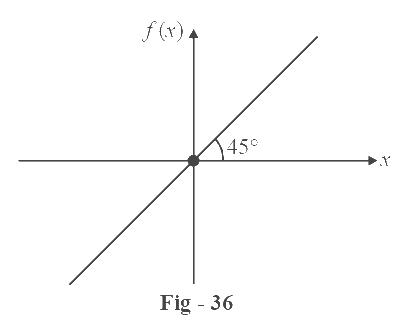D = $$\mathbb{R}$$

R = $$\mathbb{R}$$

(b) For this function, D = $$\mathbb{R}$$

\begin{align} {\text{Now }}{x^2} \ge 0\;\; \Rightarrow \quad &{x^2} + 1 \ge 1\\ \Rightarrow \quad & R = [1,\;\infty ) \end{align}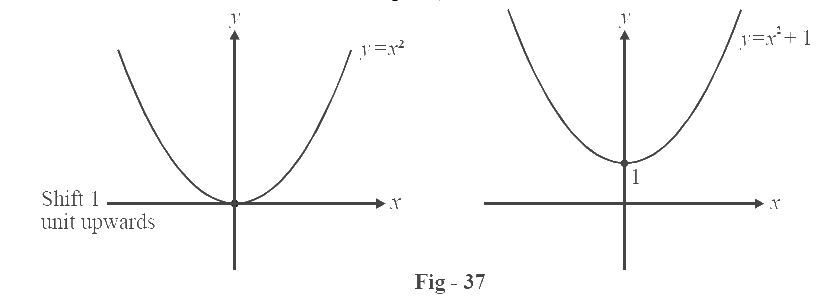We have seen the graph of y = x². The graph of  $$f(x) = {x^2} + 1$$ can be drawn by incrementing each point on the graph of $$f(x) = {x^2}$$by 1 unit along the y-axis.

(c) The argument of the root function should be non-negative.

Hence we require sin x $$\ge$$ 0.

Now we’ve seen the graph of $$f(x) = \sin x$$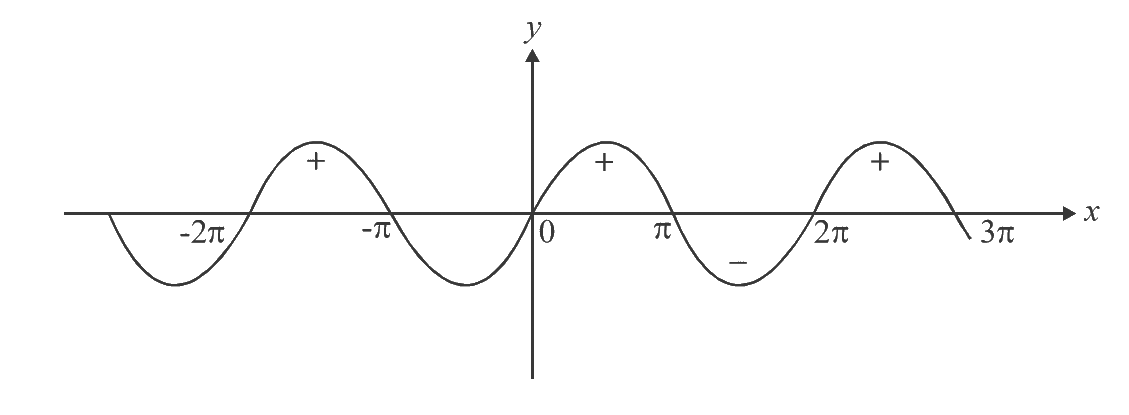sin x $$\ge$$ 0 in the intervals marked with ‘+’, i.e. [0, $$\pi$$ ], [2 $$\pi$$, 3$$\pi$$ ] and so on

D = $$\left\{ {\left[ {2n\pi ,(2n + 1)\pi } \right]} \right\},\,\,\,\,n\,\, \in \,\,\mathbb{Z}$$

For this domain, 0 < sin x < 1

$$\Rightarrow$$  $$0 \le \sqrt {\sin x} \le 1$$

$$\Rightarrow$$  $$R = [0,\,\,1]$$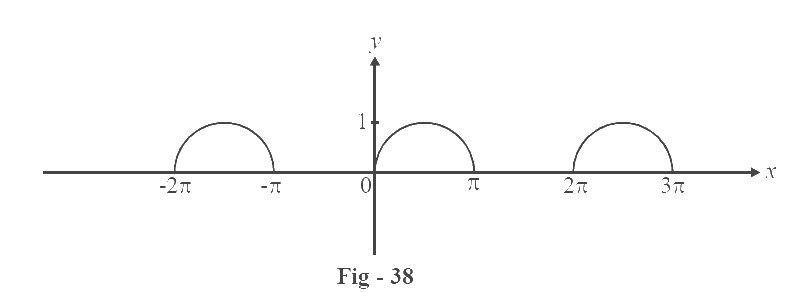(d) This function is obviously defined for all x $$\in\; \mathbb{R}$$

Therefore, D =  $$\mathbb{R}$$

Now,    –1 $$\le$$sin x $$\le$$ 1

$$\Rightarrow$$ [sin x] can take only 3 values, –1, 0 or 1.

R = {–1, 0, 1}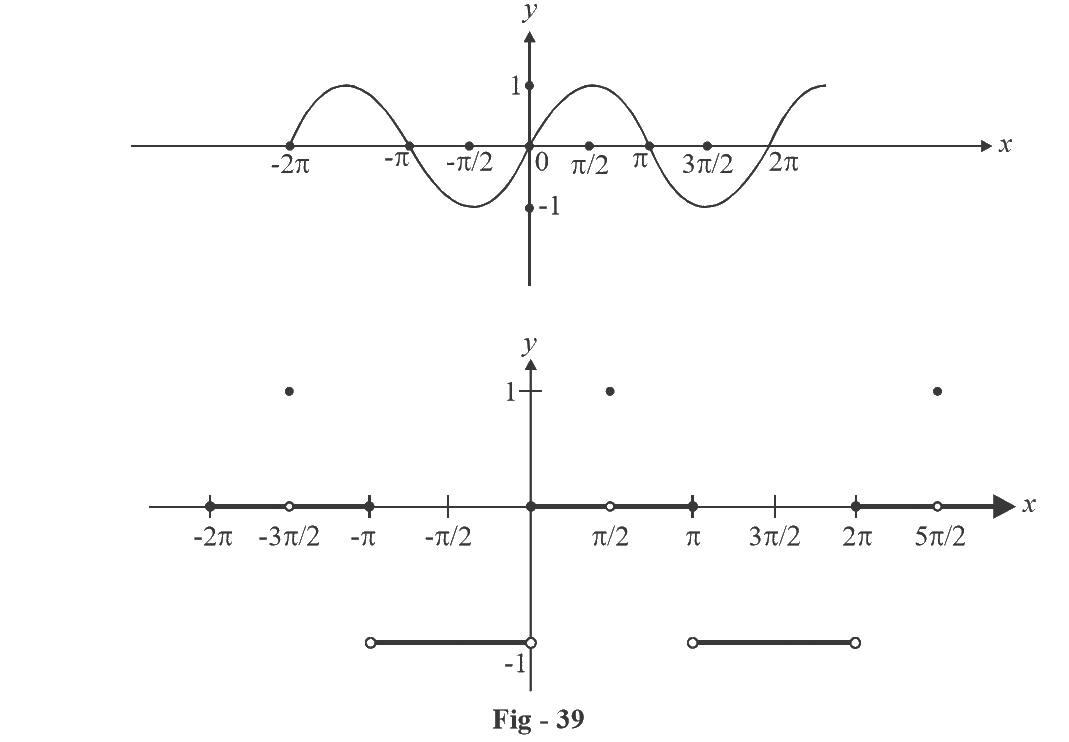(e) The denominator should never become 0.

$$\Rightarrow$$ [x] $$\ne$$ 0 $$\Rightarrow$$ x $$\notin$$ [0, 1)

Therefore, D = $$\mathbb{R}$$ – [0, 1)

To draw the graph, we note that the denominator is always a non-zero integer

Hence,\;R = \begin{align}\frac{1}{n}\end{align}\;\;where\,n \in \mathbb{Z}\, - \{ 0\} \,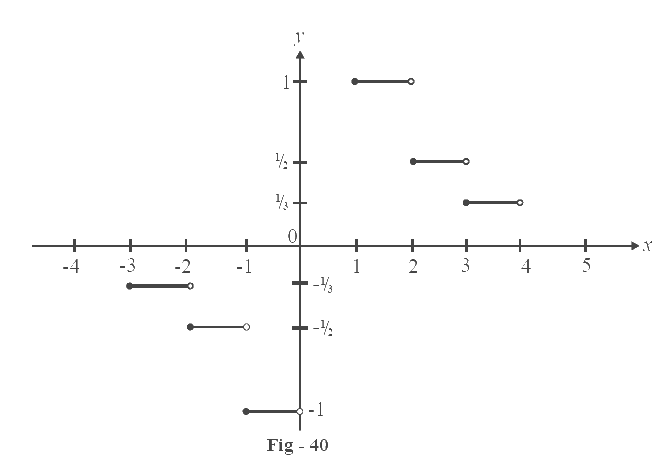(f) The domain should now be obvious.

D = $$\mathbb{R} - \{ - 1\}$$

To find the range, suppose we treat x + 1 as z, a new variable.

Then y =\begin{align}\frac{1}{z}\end{align}.

The y = \begin{align}\frac{1}{z}\end{align}graph on the y-z axis will be the same as the y = \begin{align}\frac{1}{x}\end{align}graph on the y-x axis (obviously!). `

Hence the range will be the same as the range of y =\begin{align} \frac{1}{x}\end{align}

$$\Rightarrow$$ R = $$\mathbb{R}$$– {0}

Lets draw the graph now. Consider y = \begin{align}\frac{1}{x}\end{align}.

For a particular value of x, say x = k, y = \begin{align}\frac{1}{k}\end{align}.

In the new graph y = \begin{align}\frac{1}{{x + 1}}\end{align}, we see that the same output \begin{align}\left( {y = \frac{1}{k}} \right)\end{align}, will now come 1 unit earlier, that is, at x = k – 1. This is true for all values of x. So the only difference between the two graphs will be that the new graph will be advanced (shifted to the left), by 1 unit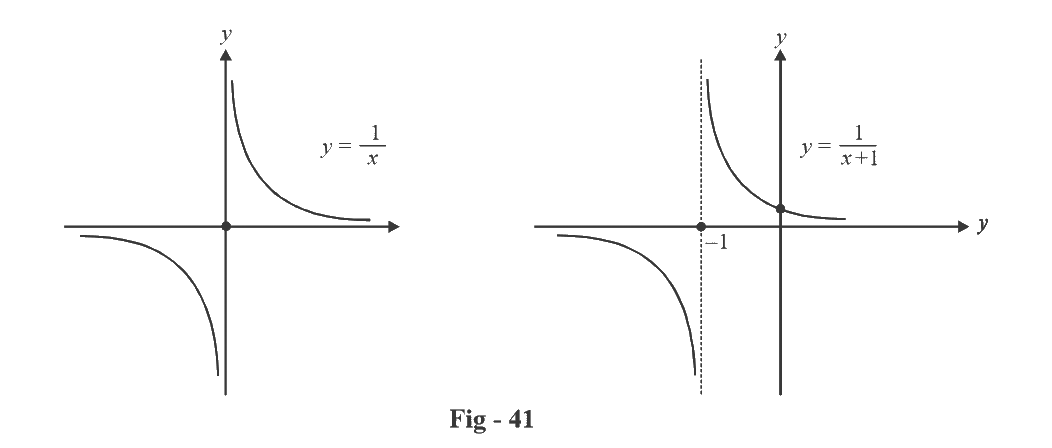By a similar logic, y =\begin{align} \frac{1}{{x - 1}}\end{align} will be delayed by 1 unit.

(g) Here, the domain has been defined explicitly as x > 0, and we see that f(x) is defined for all x > 0.

D = (0, $$\infty$$ )

To find the range (and draw the graph), lets analyse the function f (x). Suppose x > 1.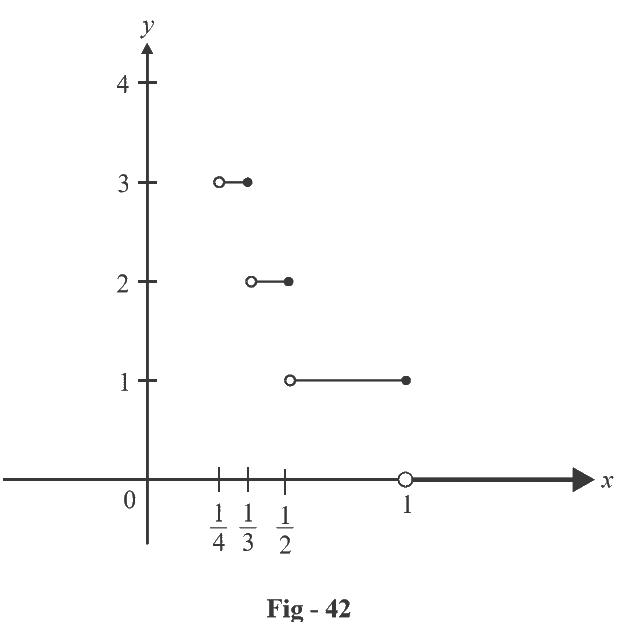Then\begin{align}\frac{1}{x} \end{align}< 1 and \left[ {\begin{align} \frac{1}{x}\end{align}} \right]= 0

Now let \begin{align}\frac{1}{2}< x <1\end{align}

$$\Rightarrow$$ 1 < \begin{align}\frac{1}{x}\end{align}< 2 and \begin{align}\left[ {\frac{1}{x}} \right]\end{align} = 1

We notice that when

\begin{align} \frac{1}{{n + 1}}\end{align} < x < \begin{align} \frac{1}{n}\end{align},

\Rightarrow n \le \begin{align}\frac{1}{x}\end{align}\, < n + 1,\,

then \begin{align}\left[ {\frac{1}{x}} \right]\end{align}= n and so on

The range is hence the non-negative integers {0, 1, 2, .......}.

The graph is now easy to draw.

(h) The function is defined for all x.

Hence D = $$\mathbb{R}$$.

Now we note that –1 < cosx < 1

$$\Rightarrow$$ 0 < | cos x | < 1

Hence, [| cos x |] can take on only two values, 0 and 1

$$\mathbb{R}$$ = {0, 1}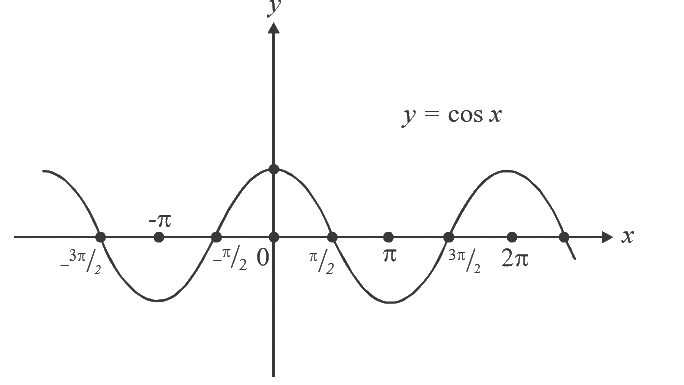The graph is obtained as shown in the figures (applying modulus function on any graph shifts the negative part into its exact mirror reflection (makes it positive of the same magnitude)).

(i) This function is again defined for all x.

D = $$\mathbb{R}$$

[cos x] has a range of {–1, 0, 1} (as in part d)

Therefore,

$$|[cos\,x]|$$has the range {0, 1}

R = {0, 1}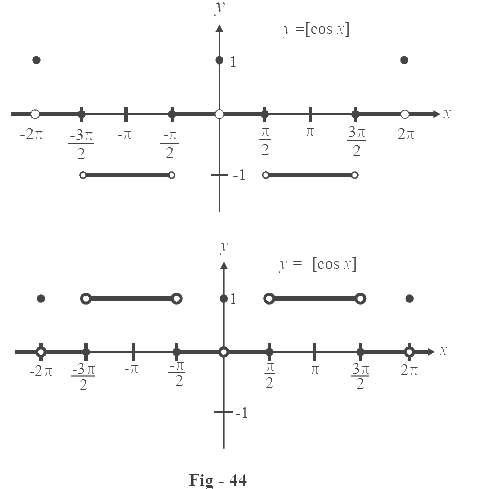The steps in obtaining the graph are shown alongside.

(j) The domain is obvious. D = $$\mathbb{R}$$

Now,

\begin{align} f(x) & = {x^2} + 3x + 2\\ & = {x^2} + 3x + \frac{9}{4} - \frac{1}{4} = {\left( {x + \frac{3}{2}} \right)^2} - \frac{1}{4} \end{align}

The first term, \begin{align}{\left( {x + \frac{3}{2}} \right)^2}\end{align} is always non-negative

$$\Rightarrow \quad {\left( {x + \frac{3}{2}} \right)^2} \ge 0$$

Hence \begin{align}f\left( x \right) = {\rm{ }}{\left( {x + \frac{3}{2}} \right)^2} - \frac{1}{4} \ge - \frac{1}{4}\end{align}

Therefore, the range is \begin{align}\left[ { - \frac{1}{4},\infty } \right)\end{align}

To draw the graph, we note that $$f\left( x \right)$$ can be obtained from $$y = {x^2}$$ in the following steps:

${x^2}\xrightarrow[{{\text{3/2 units}}}]{{{\text{Left shift}}}}{\left( {x + \frac{3}{2}} \right)^2}\xrightarrow[{{\text{1/4 units}}}]{{{\text{Down shift}}}}{\left( {x + \frac{3}{2}} \right)^2} - \frac{1}{4}$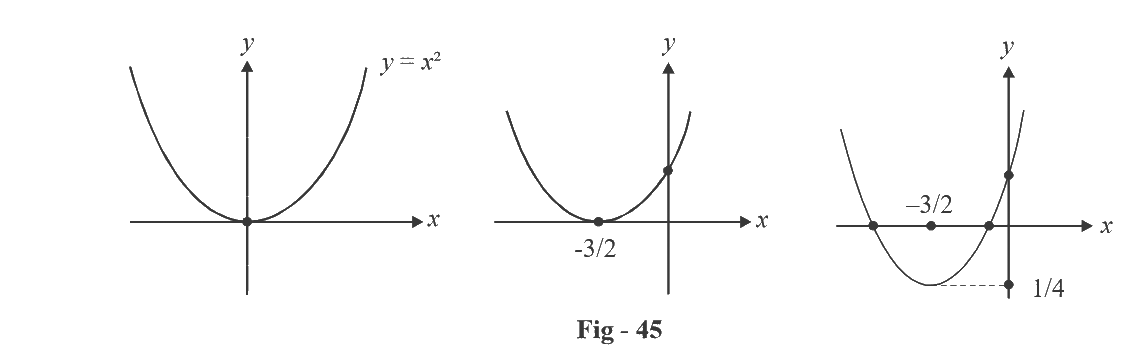The last step will become more clear in the section on graphs.